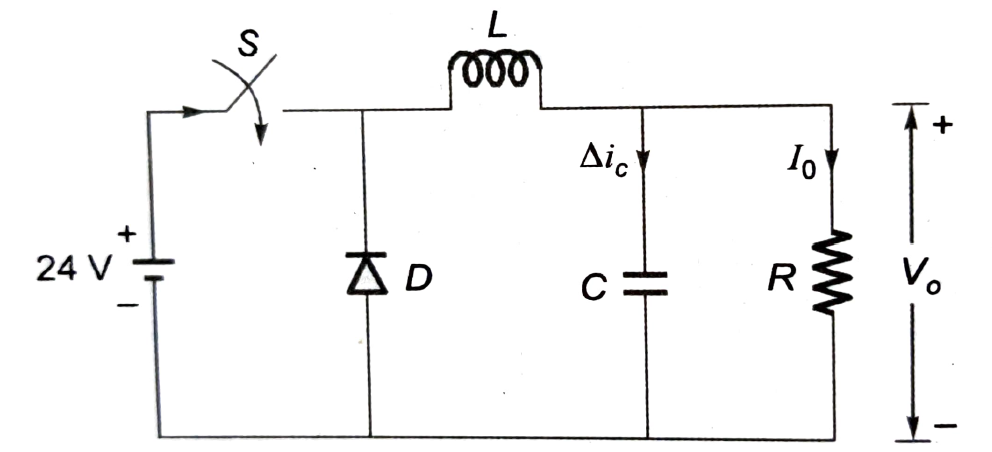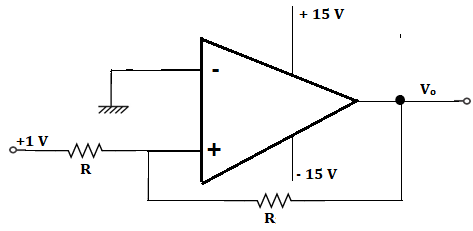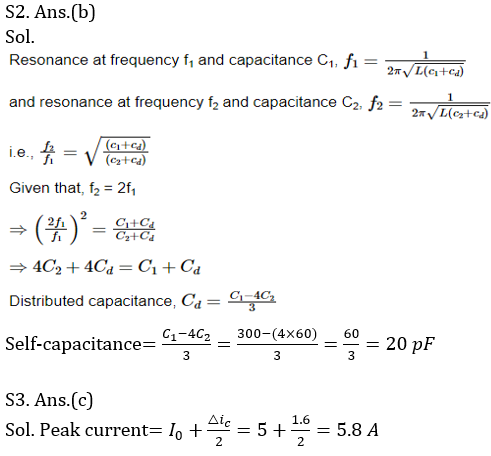Engineering Jobs   »   ELECTRICAL GATE QUIZ,

# GATE’22 EE:- Daily Practice Quiz 02-Aug-2021

Each question carries 2 marks
Negative marking: 0.66 mark

Total Questions: 05
Total marks: 10
Time: 12 min.

Q1. A 10-bus power system consists of four generator buses indexed as G_1,G_2,G_3,G_4 and six load buses indexed as L_1,L_2,L_3,L_4,L_5,L_6. The generator-bus G_1 is connected as slack bus, and the load buses L_3 and L_4 are voltage-controlled buses. The generator at bus G_2 cannot supply the required reactive power demand, and hence it is operating at its maximum reactive power limit. The number of non-linear equations required for solving the load flow problem using Newton-Raphson method in polar form is:
(a) 12
(b) 13
(c) 14
(d) 16

Q2. In a Q-meter measurement to determine the self-capacitance of a coil, the first resonance occurred at f_1 with C_1=300 pF. The second resonance occurred at f_2=2f1 with C_2=60 pF. The self-capacitance of coil works out to be:
(a) 240 pF
(b) 20 pF
(c) 360 pF
(d) 60 pF

Q3. In the circuit shown, an ideal switch S is operated at 100 kHz with a duty ratio of 50 %. Given that △i_c is 1.6 A peak to peak and I_0 is 5 A dc, the peak current in S is(a) 4.2 A
(b) 5.0 A
(c) 5.8 A
(d) 6.6 A

Q4. Slip ring motor is preferred over Squirrel Cage motor when–
1. high starting torque is required
3. heavy pull-out toque is required
(a) Only 3
(b) Only 1
(c) Only 1 and 2
(d) Only 2 and 3

Q5. In the circuit of the figure, Vo is(a) -1 V
(b) 2 V
(c) + 1 V
(d) + 15 V

SOLUTIONS

S1. Ans.(c)
Sol.
G_1: Slack bus
L_3: L_4: Voltage controlled bus (PV bus)
G_2: voltage reactive power limit (PQ bus)
PV bus = (4 – 2) + 2 = 4
PQ bus = 6 – 2 + 1 = 5
Number of non – linear equations = 2 (PQ) + PV = 2 × 5 + 4 = 14.S4. Ans.(b)
Sol. For squirrel cage induction motor, starting torque is low so when starting torque is high slip-ring induction motor is preferred because starting torque made high by connecting external resistance via slip-rings.

S5. Ans.(d)
Sol. In case of positive feedback, op-amp acts in its saturation region i.e., ± V_SAT
In this circuit, input applied voltage is positive so output voltage will also be positive.
So, V_O = 〖+V〗_SAT = +15 V

Sharing is caring!